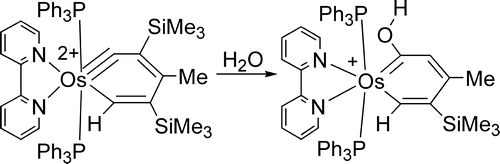# Osmabenzenes from the Reactions of a Dicationic Osmabenzyne Complex

Authors:
Wai Yiu Hung, Jun Zhu, Ting Bin Wen, Ka Po Yu, Herman H. Y. Sung, Ian D. Williams, Zhenyang Lin*, Guochen Jia*
Journal:
J. Am. Chem. Soc.
Year:
2006
Volume:
128
FirstPage-LastPage:
13742–13752
Keywords:
TOC:Abstract:

Treatment of the osmabenzyne Os(equivalent to CC(SiMe3)=C(Me)C(SiMe3)=CH)Cl-2(PPh3)(2) (1) with 2,2'-bipyridine (bipy) and thallium triflate (TlOTf) produces the thermally stable dicationic osmabenzyne [Os( equivalent to CC(SiMe3)=C(Me)C(SiMe3)=CH)(bipy)(PPh3)(2)](OTf)(2) (2). The dicationic osmabenzyne 2 reacts with ROH (R = H, Me) to give osmabenzene complexes [Os(=C(OR)CH=C(Me)C(SiMe3)=CH)(bipy)(PPh3)(2)]OTf, in which the metallabenzene ring deviates significantly from planarity. In contrast, reaction of the dicationic complex 2 with NaBH4 produces a cyclopentadienyl complex, presumably through the osmabenzene intermediate [Os(=CHC(SiMe3)=C(Me)C(SiMe3)=CH)(bipy)(PPh3)(2)] OTf. The higher thermal stability of [Os((=C(OR)CH=C(Me)C(SiMe3)=CH)(bipy)(PPh3)(2)]OTf relative to [Os(=CHC(SiMe3)=C(Me)C(SiMe3)=CH)(bipy)(PPh3) 2] OTf can be related to the stabilization effect of the OR groups on the metallacycle. A theoretical study shows that conversion of the dicationic osmabenzyne complex [Os(equivalent to CC(SiMe3)=C(Me)C(SiMe3)= CH)(bipy)( PPh3) 2](OTf) 2 to a carbene complex by reductive elimination is thermodynamically unfavorable. The theoretical study also suggests that the nonplanarity of the osmabenzenes [Os(=C(OR)CH=C(Me)C(SiMe3)=CH)(bipy)(PPh3)(2)]OTf is mainly due to electronic reasons.

Doi:
10.1021/ja064570w